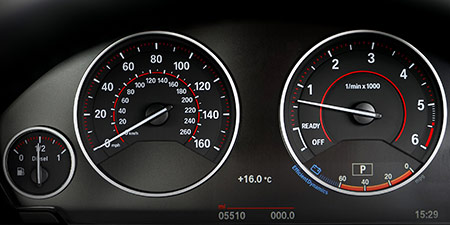# Meters per second (m/s) to Kilometers per hour (km/h)

Units of speed ﹣ Converter Meters per second to Kilometers per hour

Here you can convert the Speed unit Meters per second into the unit Kilometers per hour and vice versa you can convert Kilometers per hour into Meters per second. By clicking the "Swap units" icon, you will always obtain the desired conversion in the calculation result, i.e., m/s to km/h or km/h to m/s. With the following calculator you can also calculate any other Speed unit.

## Info about "Meters per second"

Meters per second is a basic unit for speed in the International System of Units (SI) and other metric systems. This is because both meter and second are the international base units for distance and time. Definition of "meters per second": An object moving at 1 "m/s" per second will cover a distance of one meter.

In our daily work, speed is commonly measured in km/h. The following rule is an easy way to remember when converting m/s into km/h: &qquot; multiply by four and minus 10 %". For example, standard walking speeds in meters per second are about 1 m/s for a walker, 10.44 m/s is the world record for running 100 meters, and the speed of sound is 343 meters per second.

As mentioned above, both meter and second are the international base units for distance and time. All other speed units in the metric system are derived based on meters per second (e.g. km/h, mm/s).

## Info about "Kilometers per hour"

Kilometers per hour is used as a standard unit in transportation, therefore it is used in traffic or to measure the travel speed of vehicles. For example, a commercial aircraft flies at a speed of 1,000 km/h then it covers a distance of 1,000 kilometers per hour.

The unit is defined as follows: An object moving at 1 "km/h" per hour will cover one kilometer. "km/h" is approved to use in the international system of units (SI).

## Basis for conversion Meters per second (m/s) to Kilometers per hour (km/h) and vice versa

The abbreviation for the "Speed unit Meters per second" is m/s. The abbreviation for the "Speed unit Kilometers per hour" is km/h.

## Formula for the conversion of Meters per second (m/s) to Kilometers per hour (km/h) and vice versa

The calculation from Meters per second to Kilometers per hour shall be made using the following conversion formula:

Conversion formula Meters per second to Kilometers per hour

Determine the number of Kilometers per hour from Meters per second

Meters per second × 3.6

## Formula for the conversion of Kilometers per hour (km/h) to Meters per second (m/s)

The calculation from Kilometers per hour to Meters per second shall be made using the following conversion formula:

Conversion formula Kilometers per hour to Meters per second

Determine the number of Meters per second from Kilometers per hour

Kilometers per hour × 0.27777777777778

## Overview table : How many Meters per second are in a Kilometers per hour ?

Meters per second m/s ⇒ Kilometers per hour km/h
0.01 m/s  are  0.03600 km/h
0.02 m/s  are  0.07200 km/h
0.03 m/s  are  0.10800 km/h
0.04 m/s  are  0.14400 km/h
0.05 m/s  are  0.18000 km/h
0.06 m/s  are  0.21600 km/h
0.07 m/s  are  0.25200 km/h
0.08 m/s  are  0.28800 km/h
0.09 m/s  are  0.32400 km/h
0.10 m/s  are  0.36000 km/h
0.20 m/s  are  0.72000 km/h
0.30 m/s  are  1.08000 km/h
0.40 m/s  are  1.44000 km/h
0.50 m/s  are  1.80000 km/h
0.60 m/s  are  2.16000 km/h
0.70 m/s  are  2.52000 km/h
0.80 m/s  are  2.88000 km/h
0.90 m/s  are  3.24000 km/h
1 m/s  corresponds to  3.60000 km/h
2 m/s  are  7.20000 km/h
3 m/s  are  10.80000 km/h
4 m/s  are  14.40000 km/h
5 m/s  are  18 km/h
6 m/s  are  21.60000 km/h
7 m/s  are  25.20000 km/h
8 m/s  are  28.80000 km/h
9 m/s  are  32.40000 km/h
10 m/s  are  36 km/h
20 m/s  are  72 km/h
30 m/s  are  108 km/h
40 m/s  are  144 km/h
50 m/s  are  180 km/h
60 m/s  are  216 km/h
70 m/s  are  252 km/h
80 m/s  are  288 km/h
90 m/s  are  324 km/h
100 m/s  are  360 km/h
200 m/s  are  720 km/h
300 m/s  are  1 080 km/h
400 m/s  are  1 440 km/h
500 m/s  are  1 800 km/h
600 m/s  are  2 160 km/h
700 m/s  are  2 520 km/h
800 m/s  are  2 880 km/h
900 m/s  are  3 240 km/h
1 000 m/s  are  3 600 km/h

## Overview table : How many Kilometers per hour are in a Meters per second ?

Kilometers per hour km/h ⇒ Meters per second m/s
0.01 km/h  are  0.00277 m/s
0.02 km/h  are  0.00555 m/s
0.03 km/h  are  0.00833 m/s
0.04 km/h  are  0.01111 m/s
0.05 km/h  are  0.01388 m/s
0.06 km/h  are  0.01666 m/s
0.07 km/h  are  0.01944 m/s
0.08 km/h  are  0.02222 m/s
0.09 km/h  are  0.02500 m/s
0.10 km/h  are  0.02777 m/s
0.20 km/h  are  0.05555 m/s
0.30 km/h  are  0.08333 m/s
0.40 km/h  are  0.11111 m/s
0.50 km/h  are  0.13888 m/s
0.60 km/h  are  0.16666 m/s
0.70 km/h  are  0.19444 m/s
0.80 km/h  are  0.22222 m/s
0.90 km/h  are  0.25000 m/s
1 km/h  corresponds to  0.27777 m/s
2 km/h  are  0.55555 m/s
3 km/h  are  0.83333 m/s
4 km/h  are  1.11111 m/s
5 km/h  are  1.38888 m/s
6 km/h  are  1.66666 m/s
7 km/h  are  1.94444 m/s
8 km/h  are  2.22222 m/s
9 km/h  are  2.50000 m/s
10 km/h  are  2.77777 m/s
20 km/h  are  5.55555 m/s
30 km/h  are  8.33333 m/s
40 km/h  are  11.11111 m/s
50 km/h  are  13.88888 m/s
60 km/h  are  16.66666 m/s
70 km/h  are  19.44444 m/s
80 km/h  are  22.22222 m/s
90 km/h  are  25 m/s
100 km/h  are  27.77777 m/s
200 km/h  are  55.55555 m/s
300 km/h  are  83.33333 m/s
400 km/h  are  111.11111 m/s
500 km/h  are  138.88888 m/s
600 km/h  are  166.66666 m/s
700 km/h  are  194.44444 m/s
800 km/h  are  222.22222 m/s
900 km/h  are  250 m/s
1 000 km/h  are  277.77777 m/s

## Source information

As source for the information in the 'Units of speed' category, we have used in particular:

## Last update

This page of the 'Units of speed' category was last edited or reviewed by Stefan Banse on February 19, 2023. It corresponds to the current status.

### Changes in this category "Units of speed"

• November 9, 2020: Publication of the Speed converter